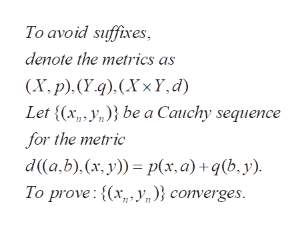# 2. Assume that (X, dX) and (Y, dY ) are complete spaces, and give X × Y themetric d defined byd((x1, y1),(x2, y2)) = dX(x1, x2) + dY (y1, y2)Show that (X × Y, d) is complete.

Question
10 views

2. Assume that (X, dX) and (Y, dY ) are complete spaces, and give X × Y the
metric d defined by
d((x1, y1),(x2, y2)) = dX(x1, x2) + dY (y1, y2)
Show that (X × Y, d) is complete.

check_circle

Step 1

To prove that every Cauchy sequence in the product space XxY converges (under the metric defined in the problem)

Step 2

Statement to be provedhelp_outlineImage TranscriptioncloseTo avoid suffixes denote the metrics as (X.p). (Y.4). (Xx Y, d Let {(x, ybe a Cauchy sequence for the metric d(a,b).(x, y) p(x, a) + q(b, y). To prove:(xy)} converges fullscreen
Step 3

First prove that the projections of any Cauchy sequenc...

### Want to see the full answer?

See Solution

#### Want to see this answer and more?

Solutions are written by subject experts who are available 24/7. Questions are typically answered within 1 hour.*

See Solution
*Response times may vary by subject and question.
Tagged in

### Math